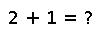# one

one(x)

Get the multiplicative identity element for the type of `x` (`x` can also specify the type itself). For matrices, returns an identity matrix of the appropriate size and type.

## Examples

``````julia> one(10)
1
julia> foo = zeros(Int8, (2,2));
julia> one(foo)
2x2 Array{Int8,2}:
1  0
0  1
julia> complexNumber = complex(1, 1);   # 1 + 1im
julia> one(complexNumber)
1 + 0im``````

Checking you are not a robot: